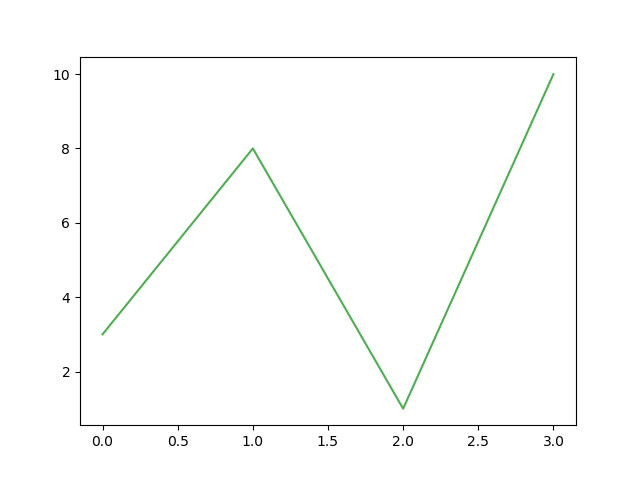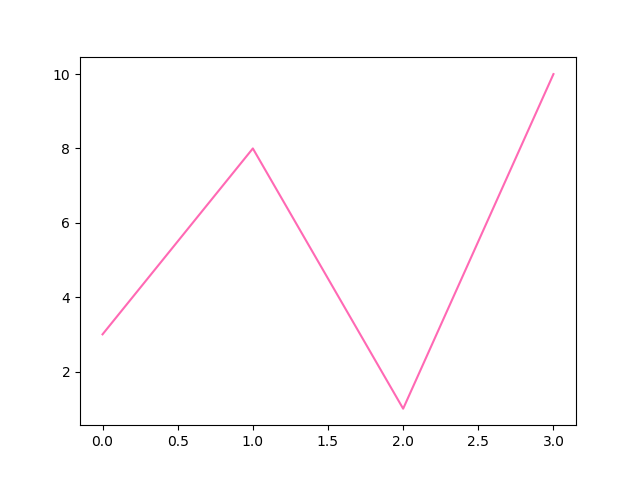# Matplotlib Line

## Linestyle

You can use the keyword argument `linestyle`, or shorter `ls`, to change the style of the plotted line:

### Example

Use a dotted line:

### Result:Try it Yourself »

### Example

Use a dashed line:

### Result:Try it Yourself »

Shorter Syntax

The line style can be written in a shorter syntax:

`linestyle` can be written as `ls`.

`dotted` can be written as `:`.

`dashed` can be written as `--`.

Shorter syntax:

### Result:Try it Yourself »

## Line Styles

You can choose any of these styles:

Style Or
‘solid’ (default) ‘-‘ Try it »
‘dotted’ ‘:’ Try it »
‘dashed’ ‘–‘ Try it »
‘dashdot’ ‘-.’ Try it »
‘None’ ” or ‘ ‘ Try it »

## Line Color

You can use the keyword argument `color` or the shorter `c` to set the color of the line:

### Example

Set the line color to red:

### Result:Try it Yourself »

You can also use Hexadecimal color values:

### Example

Plot with a beautiful green line:

### Result:Try it Yourself »

Or any of the 140 supported color names.

### Example

Plot with the color named “hotpink”:

plt.plot(ypoints, c = ‘hotpink’)

### Result:Try it Yourself »

## Line Width

You can use the keyword argument `linewidth` or the shorter `lw` to change the width of the line.

The value is a floating number, in points:

### Example

Plot with a 20.5pt wide line:

import matplotlib.pyplot as plt
import numpy as np

ypoints = np.array()

plt.plot(ypoints, linewidth = ‘20.5’)
plt.show()

### Result:Try it Yourself »

## Multiple Lines

You can plot as many lines as you like by simply adding more `plt.plot()` functions:

### Example

Draw two lines by specifying a `plt.plot()` function for each line:

import matplotlib.pyplot as plt
import numpy as np

y1 = np.array()
y2 = np.array()

plt.plot(y1)
plt.plot(y2)

plt.show()

### Result:Try it Yourself »

You can also plot many lines by adding the points for the x- and y-axis for each line in the same `plt.plot()` function.

(In the examples above we only specified the points on the y-axis, meaning that the points on the x-axis got the the default values (0, 1, 2, 3).)

The x- and y- values come in pairs:

### Example

Draw two lines by specifiyng the x- and y-point values for both lines:

import matplotlib.pyplot as plt
import numpy as np

x1 = np.array()
y1 = np.array()
x2 = np.array()
y2 = np.array()

plt.plot(x1, y1, x2, y2)
plt.show()

### Result:Try it Yourself »